# Workshopping some Plots

Today’s R Club will focus on workshopping one straightforward data wrangling task, and plotting data from Dani’s and Arian’s Intentional Regulation of Craving study.

• Use the imageCats.csv to create columns for the image category and image number (i.e. if choices are 1=chocolate, 2=donuts, then first01.jpg –> chocolate01, second07.jpg –> donuts07, etc.).
• Use new image info to further explore the data

Check out past posts on ggplot (especially the ones at the bottom) to get some help on plotting. You also might want to read about the philosophy of ggplot. The diagram below may also help you get a sense for how ggplot works.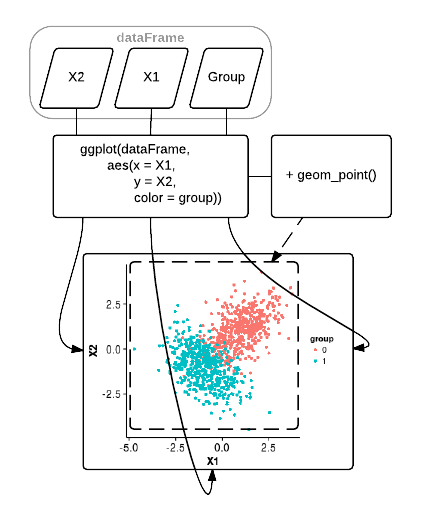``````library(dplyr)
library(tidyr)
library(ggplot2)
library(psych)
library(knitr)``````

# Import

``````Data = read.csv("FP_DataTable.csv", header=TRUE);

# Tidy

``````# Recode missing data
Data[Data == "NaN"] = "NA";

# Set variable types
Data\$ReportResp <- as.factor(Data\$ReportResp);
levels(Data\$ReportResp) <- c("look", "regulate", "NA");

Data\$RateResp <- as.numeric(Data\$RateResp);
Data\$RateRT <- as.numeric(Data\$RateRT);
Data\$RatingResp <- as.numeric(Data\$RatingResp);
Data\$RatingRT <- as.numeric(Data\$RatingRT);

# Recode button box responses
Data <- Data %>% mutate(RateResp = RateResp-4)

# Define conditions
Data <- Data %>% mutate(Condition=(paste0(Instruction, ReportResp)))

Data\$Condition[Data\$Condition == "looklook"] = "cued_look";
Data\$Condition[Data\$Condition == "regulateregulate"] = "cued_regulate";
Data\$Condition[Data\$Condition == "chooselook"] = "int_look";
Data\$Condition[Data\$Condition == "chooseregulate"] = "int_regulate";

# Recode NA trials
Data\$Condition[Data\$Condition == "chooseNA"] = "NA"
Data\$Condition[Data\$Condition == "lookNA"] = "NA"
Data\$Condition[Data\$Condition == "regulateNA"] = "NA"
Data\$Condition[Data\$Condition == "lookregulate"] = "NA"
Data\$Condition[Data\$Condition == "regulatelook"] = "NA"

# Set Condition as a factor
Data\$Condition = as.factor(Data\$Condition)``````

# Descriptives

``````# get rid of NA trials so that only cued_look, cued_regulate, int_look, int_regulate are left
Data1 = Data %>% filter(grepl("int|cued", Condition))

# get ns for each condition
Data1 %>% group_by(Condition) %>% count(Condition)``````
``````## Source: local data frame [4 x 2]
##
##       Condition     n
##          (fctr) (int)
## 1     cued_look   654
## 2 cued_regulate   646
## 3      int_look   682
## 4  int_regulate   660``````
``````# for each subject, get the number of intentional look and regulates
conditionNs <- Data1 %>% group_by(SubjectID) %>% count(SubjectID, Condition) %>% filter(grepl('^int', Condition))

# graph boxplot of each condition
p <- ggplot(conditionNs, aes(factor(Condition), n))
p + geom_boxplot()``````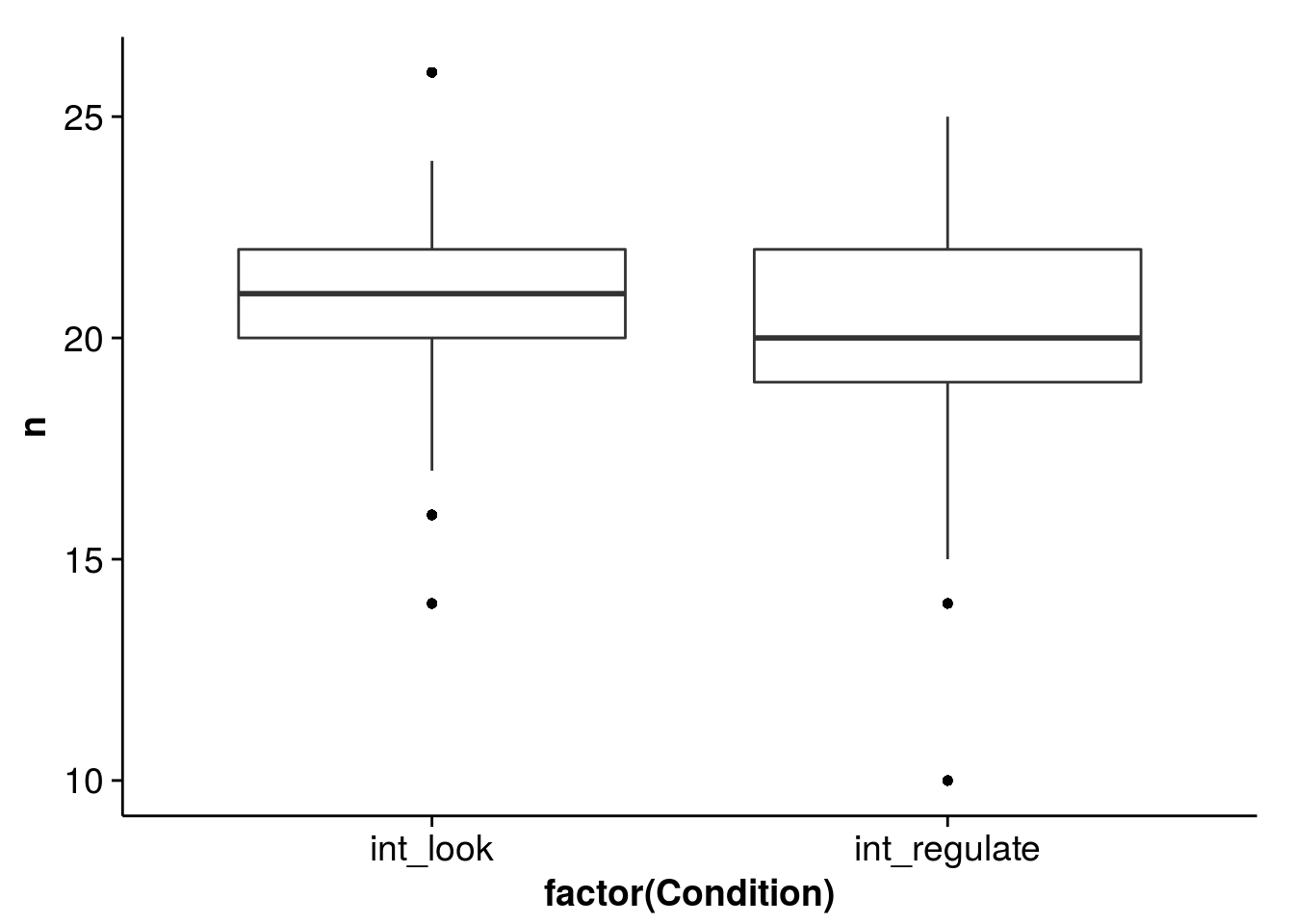``````# compute ratio of intentional look to intentional regulate trials
ratioNs <- conditionNs %>% group_by(SubjectID,Condition) %>% spread(Condition,n) %>% mutate(ratio = int_look/int_regulate)

# plot the distribution of ratios
p <- ggplot(ratioNs, aes(x=ratio))
p + geom_histogram(binwidth=.1, aes(fill = ..count..)) +
labs(title = "Distribution of ratios of intentional look trials to intentional regulate trials")``````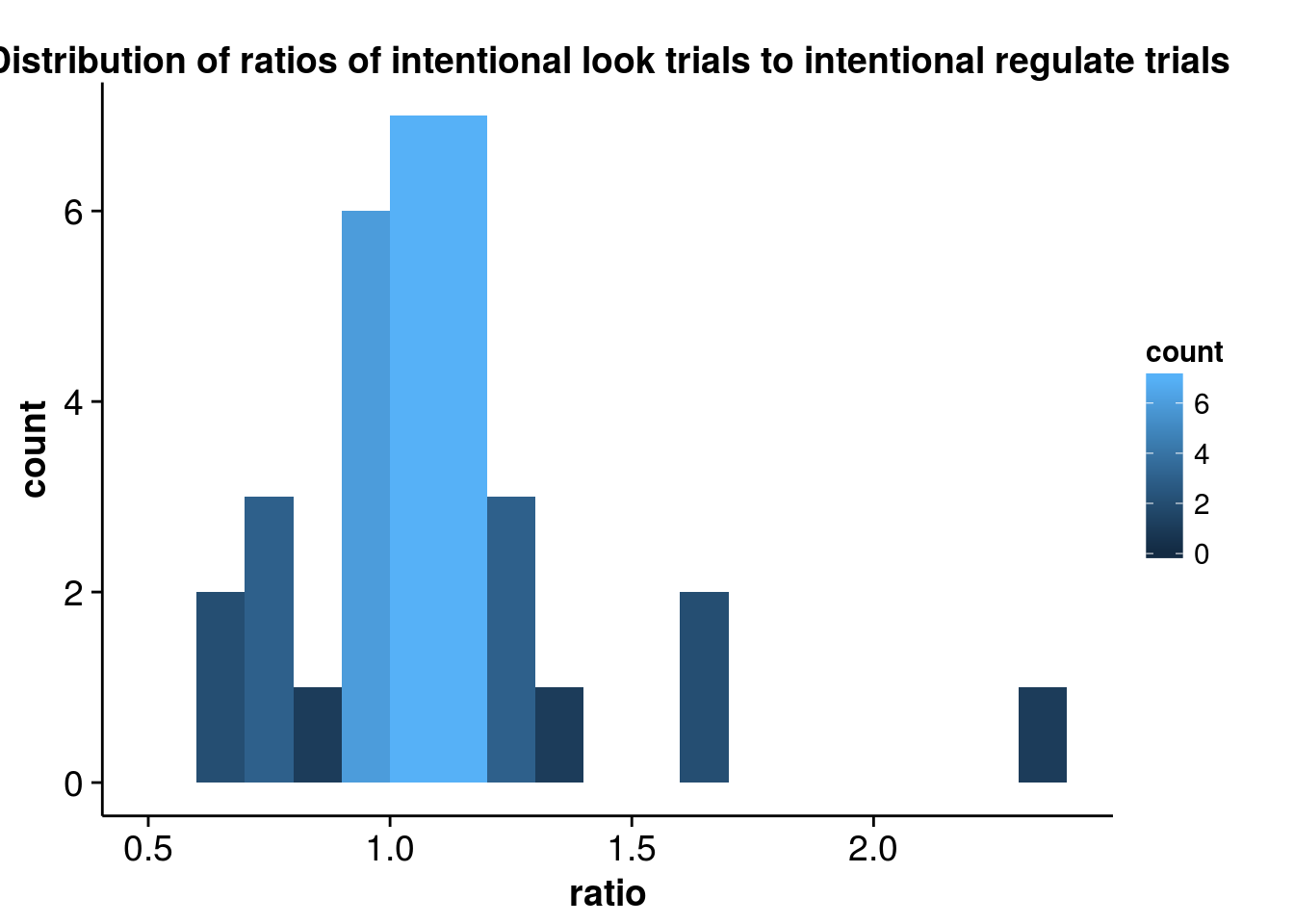``````# summarize data
describeBy(Data1\$RateResp, Data1\$Condition)``````
``````## group: cued_look
##   vars   n mean  sd median trimmed  mad min max range  skew kurtosis   se
## 1    1 651 3.69 1.2      4    3.83 1.48   1   5     4 -0.69    -0.45 0.05
## --------------------------------------------------------
## group: cued_regulate
##   vars   n mean   sd median trimmed  mad min max range skew kurtosis   se
## 1    1 645  2.4 1.06      2    2.32 1.48   1   5     4 0.61    -0.19 0.04
## --------------------------------------------------------
## group: int_look
##   vars   n mean   sd median trimmed  mad min max range  skew kurtosis   se
## 1    1 681 3.59 1.19      4     3.7 1.48   1   5     4 -0.57    -0.58 0.05
## --------------------------------------------------------
## group: int_regulate
##   vars   n mean   sd median trimmed  mad min max range skew kurtosis   se
## 1    1 655 2.45 1.02      2     2.4 1.48   1   5     4 0.43    -0.35 0.04
## --------------------------------------------------------
## group: NA
## NULL``````
``````# boxplots for task ratings by condition
p <- ggplot(Data1, aes(factor(Condition), RateResp))
p + geom_boxplot()``````
``## Warning: Removed 10 rows containing non-finite values (stat_boxplot).``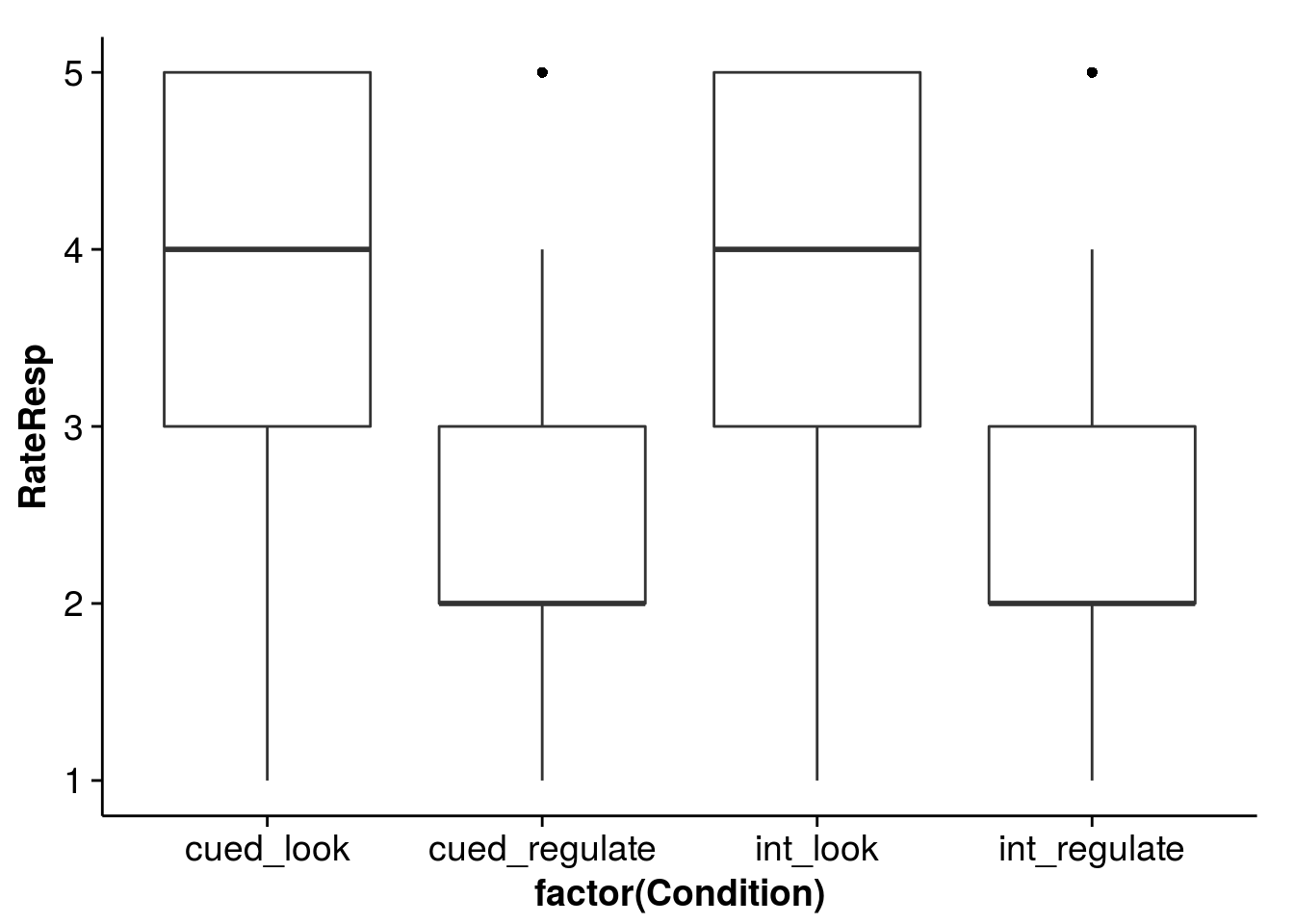``````# histograms for task ratings by condition
p <- ggplot(Data1, aes(x=RateResp))
p + geom_histogram(binwidth=1, aes(fill = ..count..)) +
xlim(1, 5) +
facet_wrap(~ Condition, nrow = 2) +
labs(title = "Distribution of trials")``````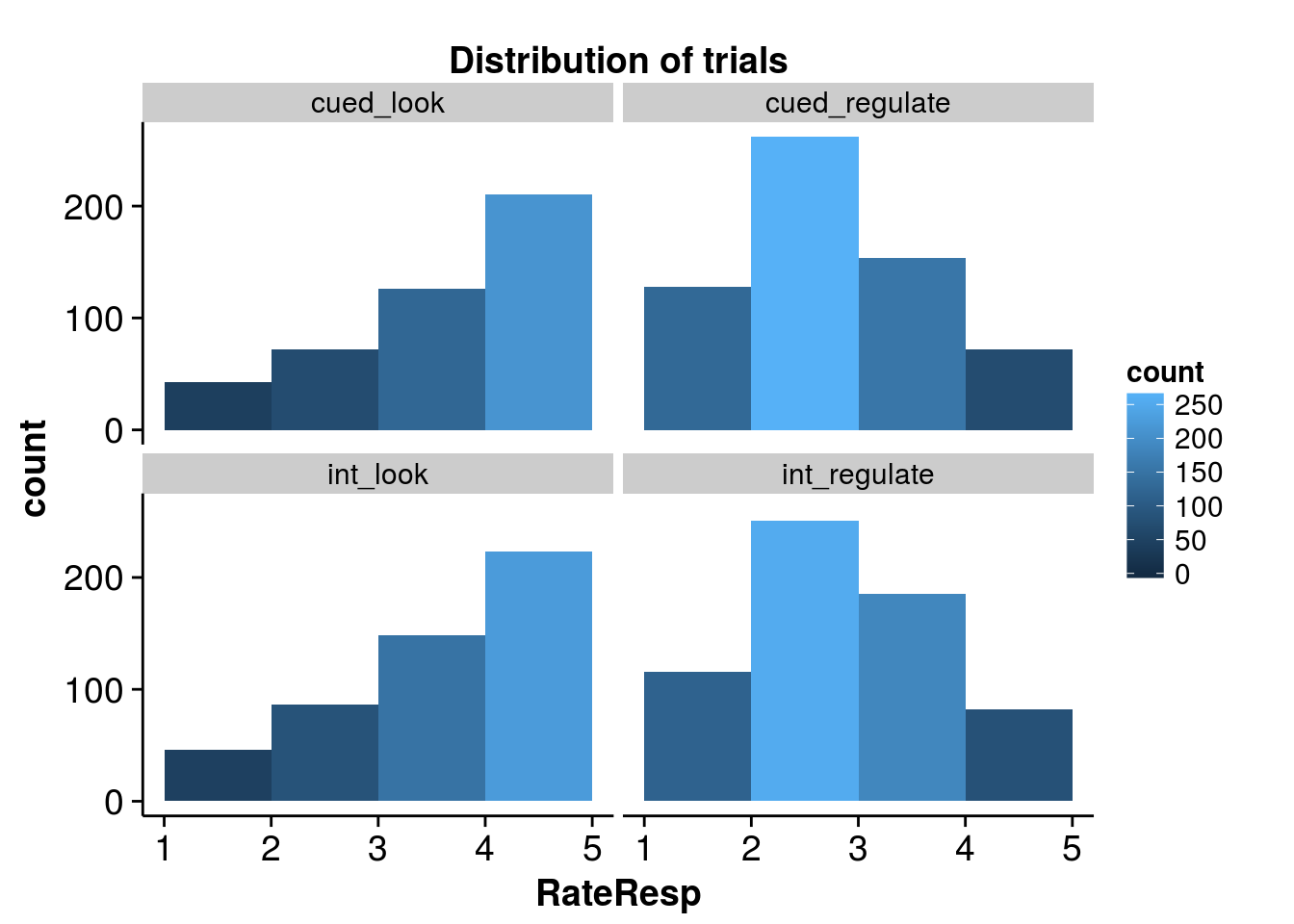``````# create plot.rate data frame for task ratings
plot.rate = Data1 %>% group_by(Condition) %>% summarise(meanRateResp = mean(RateResp, na.rm=TRUE), sd = sd(RateResp, na.rm=TRUE), n = n(), sem=sd(RateResp, na.rm=TRUE)/sqrt(n), ci=qt(0.975,df=n-1)*sem)

plot.rate\$Cue = c("cued", "cued", "intentional", "intentional")
plot.rate\$Condition = c("look", "regulate","look","regulate")
plot.rate\$Condition = as.factor(plot.rate\$Condition)

# create plot.rt data frame for reaction times
plot.rt = Data1 %>% group_by(Condition) %>% summarise(meanRT = mean(RateRT, na.rm=TRUE), sd = sd(RateRT, na.rm=TRUE), n = n(), sem=sd(RateRT, na.rm=TRUE)/sqrt(n), ci=qt(0.975,df=n-1)*sem)

plot.rt\$Cue = c("cued", "cued", "intentional", "intentional")
plot.rt\$Condition = c("look", "regulate","look","regulate")
plot.rt\$Condition = as.factor(plot.rt\$Condition)

# create plot.rate data frame for post-task ratings
plot.rating = Data1 %>% group_by(Condition) %>% summarise(meanRating = mean(RatingResp, na.rm=TRUE), sd = sd(RatingResp, na.rm=TRUE), n = n(), sem=sd(RatingResp, na.rm=TRUE)/sqrt(n), ci=qt(0.975,df=n-1)*sem) %>% filter(Condition != 'NA')

plot.rating\$Cue = c("cued", "cued", "intentional", "intentional")
plot.rating\$Condition = c("look", "regulate","look","regulate")
plot.rating\$Condition = as.factor(plot.rating\$Condition)

# plot mean RateResp by Condition with error bars
limits=aes(ymax=plot.rate\$meanRateResp + ci, ymin=plot.rate\$meanRateResp - ci)
dodge = position_dodge(width=0.1)

p = ggplot(plot.rate, aes(x=Condition, y=meanRateResp, colour=Cue))
p + geom_line(aes(group=Cue), position=dodge, size=1.5) +
geom_errorbar(limits, position=dodge, width=0.05, size=1.5) +
ylab("Mean Rating")``````
``## ymax not defined: adjusting position using y instead``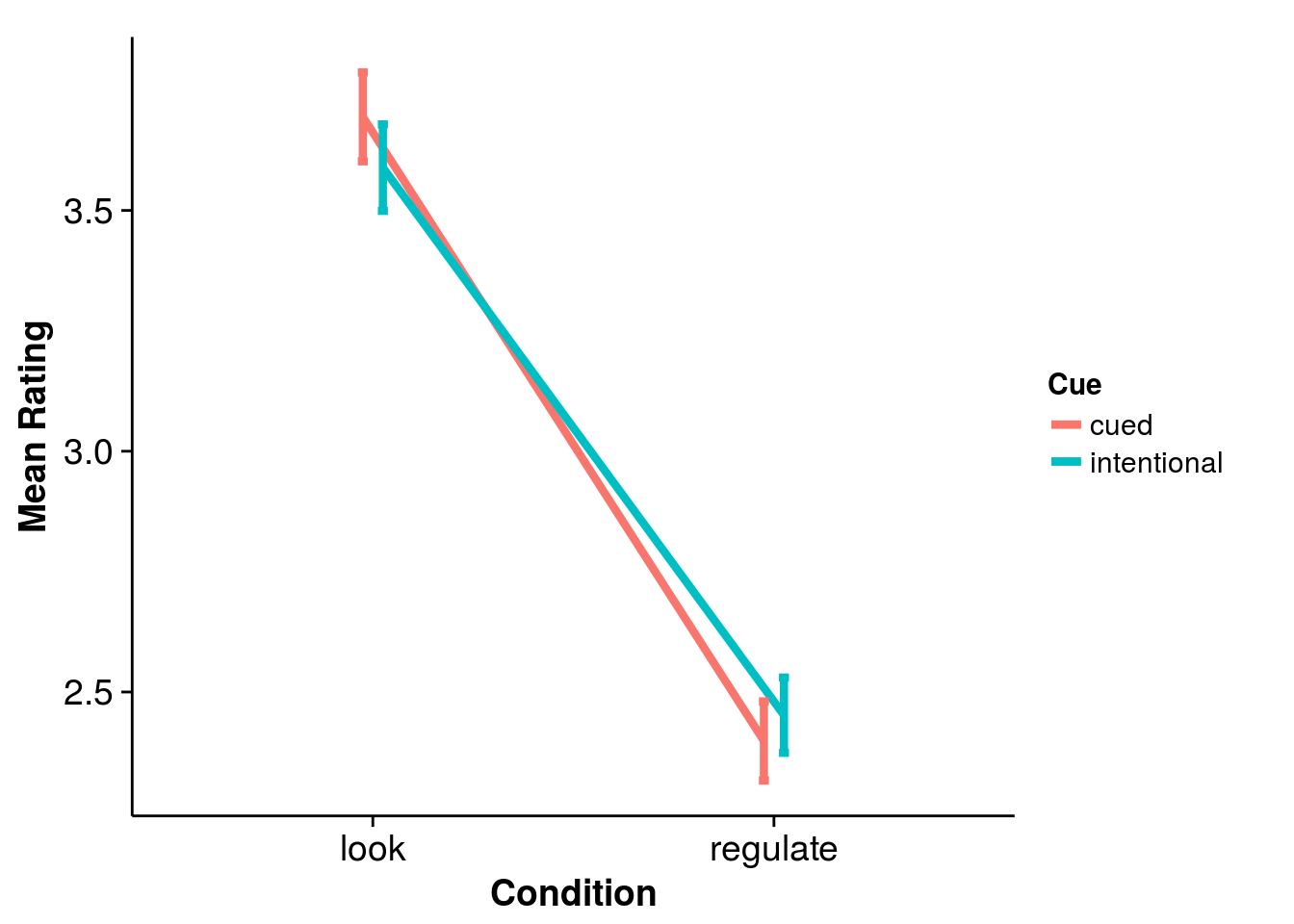``````# plot mean RateRT by Condition with error bars
limits=aes(ymax=plot.rt\$meanRT + ci, ymin=plot.rt\$meanRT - ci)
dodge = position_dodge(width=0.1)

p = ggplot(plot.rt, aes(x=Condition, y=meanRT, colour=Cue))
p + geom_line(aes(group=Cue), position=dodge, size=1.5) +
geom_errorbar(limits, position=dodge, width=0.05, size=1.5) +
ylab("Mean Rating RT") ``````
``## ymax not defined: adjusting position using y instead``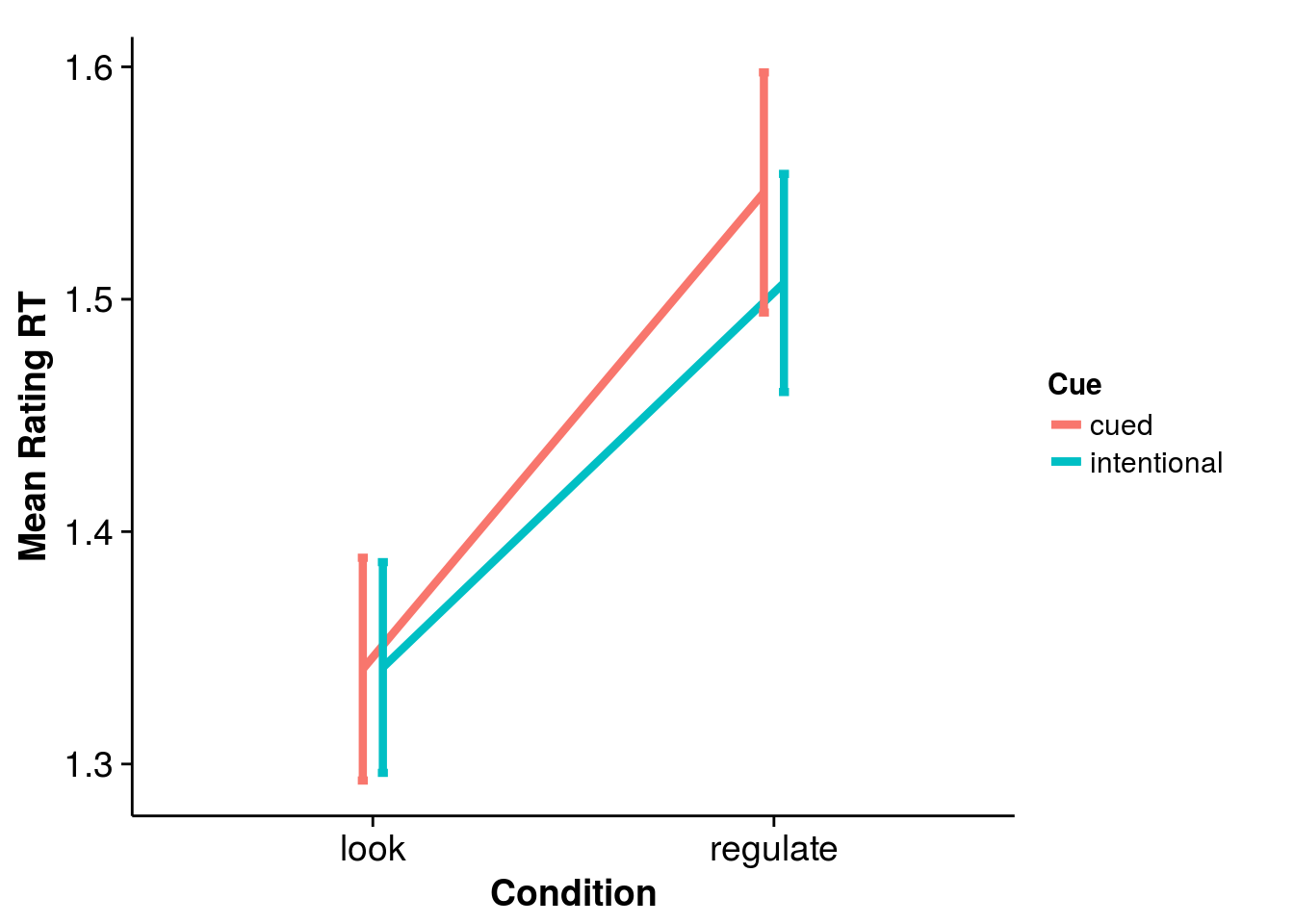``````# plot mean Rating by Condition with error bars
limits=aes(ymax=plot.rating\$meanRating + ci, ymin=plot.rating\$meanRating - ci)
dodge = position_dodge(width=0.1)

p = ggplot(plot.rating, aes(x=Condition, y=meanRating, colour=Cue))
p + geom_line(aes(group=Cue), position=dodge, size=1.5) +
geom_errorbar(limits, position=dodge, width=0.05, size=1.5) +
``## ymax not defined: adjusting position using y instead``